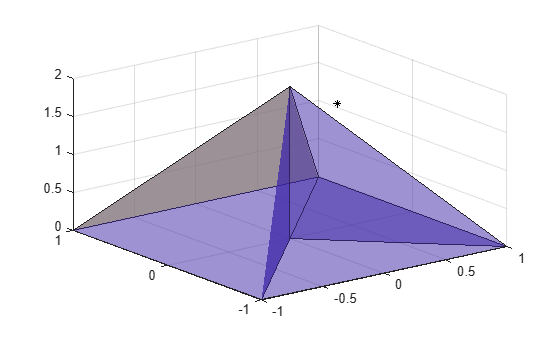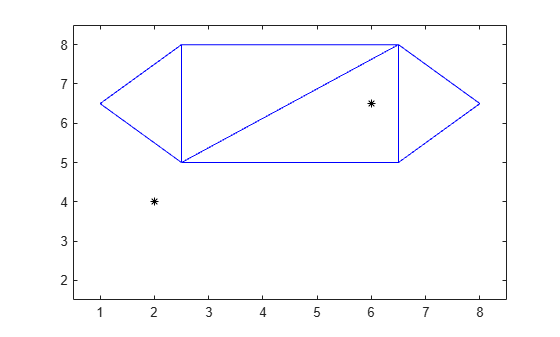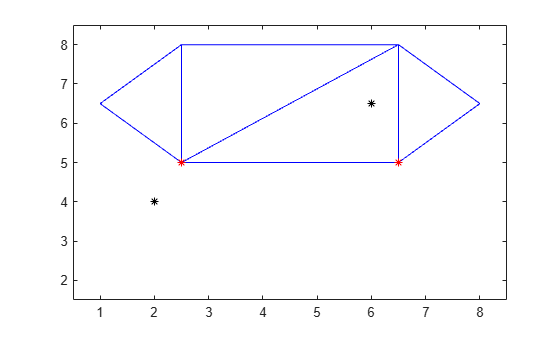# nearestNeighbor

Vertex closest to specified point

## Syntax

``ID = nearestNeighbor(TR,P)``
``ID = nearestNeighbor(TR,x,y)``
``ID = nearestNeighbor(TR,x,y,z)``
``````[ID,d] = nearestNeighbor(___)``````

## Description

example

````ID = nearestNeighbor(TR,P)` returns the IDs of the vertices closest to the query points in `P`. Each row in the matrix `P` contains the coordinates of a query point.```
````ID = nearestNeighbor(TR,x,y)` specifies the `x`-coordinates and `y`-coordinates of 2-D query points as separate column vectors.```

example

````ID = nearestNeighbor(TR,x,y,z)` specifies the x-coordinates, y-coordinates, and z-coordinates of 3-D query points as separate column vectors.```

example

``````[ID,d] = nearestNeighbor(___)``` also returns the Euclidean distance between each query point and its nearest neighbor for any of the previous syntaxes.```

## Examples

collapse all

Compute the nearest neighbors in a 3-D triangulation.

Create a 3-D Delaunay triangulation.

```P = [1 1 0; -1 1 0; -1 -1 0; 1 -1 0; 0 0 2; 0 0 0]; TR = delaunayTriangulation(P);```

Plot the triangulation and a query point.

```tri = TR(:,:); trisurf(tri,P(:,1),P(:,2),P(:,3),'FaceAlpha',0.5) hold on x = 0; y = -0.5; z = 2; plot3(x,y,z,'k*')```Find the coordinates of the nearest neighbor to the query point.

```ID = nearestNeighbor(TR,x,y,z); C = TR.Points(ID,:)```
```C = 1×3 0 0 2 ```

Compute the nearest neighbors in a 2-D triangulation.

Create a 2-D triangulation.

```C = [5 3 1; 3 2 1; 3 4 2; 4 6 2]; TP = [2.5 8.0; 6.5 8.0; 2.5 5.0; 6.5 5.0; 1.0 6.5; 8.0 6.5]; TR = triangulation(C,TP);```

Define two query points.

`P = [2 4; 6 6.5];`

Plot the triangulation and the query points.

```triplot(TR) hold on plot(P(:,1),P(:,2),'k*') ylim([1.5 8.5]) xlim([0.5 8.5])```Find the nearest neighbors to the query points and the distances between them.

`[ID,d] = nearestNeighbor(TR,P);`

Highlight in red the points in the triangulation that are the nearest neighbors to the query points.

```N = TP(ID,:); plot(N(:,1),N(:,2),'*r')```Display the distance between each query point and its nearest neighbor.

`d`
```d = 2×1 1.1180 1.5811 ```

## Input Arguments

collapse all

Triangulation representation, specified as a scalar `triangulation` or `delaunayTriangulation` object. `nearestNeighbor` does not support `delaunayTriangulation` objects with constrained edges.

Data Types: `triangulation` | `delaunayTriangulation`

Query points, specified as a matrix with 2 or 3 columns. `P` contains the x-coordinates, y-coordinates, and (possibly) z-coordinates of the query points.

Data Types: `double`

x-coordinates of query points, specified as a column vector.

Data Types: `double`

y-coordinates of query points, specified as a column vector.

Data Types: `double`

z-coordinates of query points, specified as a column vector.

Data Types: `double`

## Output Arguments

collapse all

Vertex IDs of the nearest neighbors to the query points, returned as a column vector. A vertex ID is the row number of the corresponding vertex in the `Points` property.

Data Types: `double`

Euclidean distances from the query points to their nearest neighbors, returned as a column vector the same length as `ID`.

Data Types: `double`